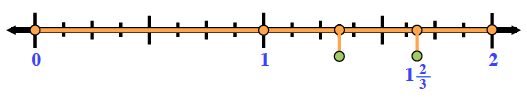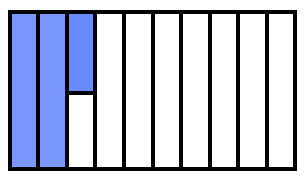### Home > CC1MN > Chapter 8 > Lesson 8.2.4 > Problem8-108

8-108.

Represent each of the following numbers two ways: by drawing an area model and by using a number line.

1. $1\frac { 2 } { 3 }$

• Use the number line below to help you draw a diagram.1. $125\%$